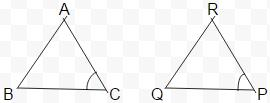Courses

# Test: Congruence Of Triangles - 1

## 10 Questions MCQ Test Mathematics (Maths) Class 7 | Test: Congruence Of Triangles - 1

Description
This mock test of Test: Congruence Of Triangles - 1 for Class 7 helps you for every Class 7 entrance exam. This contains 10 Multiple Choice Questions for Class 7 Test: Congruence Of Triangles - 1 (mcq) to study with solutions a complete question bank. The solved questions answers in this Test: Congruence Of Triangles - 1 quiz give you a good mix of easy questions and tough questions. Class 7 students definitely take this Test: Congruence Of Triangles - 1 exercise for a better result in the exam. You can find other Test: Congruence Of Triangles - 1 extra questions, long questions & short questions for Class 7 on EduRev as well by searching above.
QUESTION: 1

### Two angles are congruent if they have

Solution:

When 2 angles are equal in measurement, they are said to be congruent.

QUESTION: 2

### Which of the following is not a congruence criterion?

Solution:

We have ASA ,SSS,SAS and RHS are congruence criterions.

QUESTION: 3

### Which angle is included between the sides DE and EF of △DEF?

Solution:

When you join the line DE and EF you will find a common vertex E , which is the included angle.

QUESTION: 4

In ΔABC and ΔPQR, AB = 4 cm, BC = 5 cm, AC = 6 cm and PQ = 4 cm. QR = 5 cm. PR = 6 cm. then which of the following is true?

Solution:

In the triangles we have AB=PQ,BC=QR and AC=PR , so we write the name of the triangles such that the corresponding sides are equal, ie, AB=PQ so it will beABC ≅ PQR

QUESTION: 5

If ΔDEF ≅ ΔBCA, then the part of ΔBCA that correspond to ∠E is

Solution:

In a congruence , triangles’ name are written such that the corresponding angles of both the triangles are equal, meaning that ∠D=∠B,∠E=∠C and ∠F=∠A. So , ∠C is the correct answer.

QUESTION: 6

If ΔDEF ≅ ΔACB, then the part of ΔACB that correspond to ∠F is

Solution:

In a congruence , triangles’ name are written such that the corresponding angles of both the triangles are equal, meaning that ∠D=∠A,∠E=∠C and ∠F=∠B. So , ∠B is the correct answer.

QUESTION: 7

ΔABC and ΔPQR are congruent under the correspondence: ABC ↔ RQP, then the part of ΔABC that correspond to ∠P is

Solution:From above diagram, it is clear that ∠C corresponds to ∠P

QUESTION: 8

ΔABC and ΔPQR are congruent under the correspondence: ABC ↔ RQP, then the part of ΔABC that correspond to ∠Q is

Solution:
QUESTION: 9

ΔABC and ΔPQR are congruent under the correspondence: ABC ↔ RPQ, then the part of ΔABC that correspond to PQ is

Solution:
QUESTION: 10

What is the side included between the angles A and C of △ABC?

Solution: